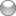# Digital precision glitch

4 replies [Last post]
jabatesOffline
Joined: 10/15/2009
Posts:

I found that if I entered the equation pi / 2 = it gives 1.570796326794896619231321691639751. But, if I enter pi / (sqrt(4)) =, the result is 1.5707963267949, dropping off more than half the digital precision. Since sqrt (4) = 2, there shouldn't be a drop in the accuracy. Likewise, sqrt(2) = 1.4142135623731, and entering pi / 1.4142135623731 gives 2.221441469079175346183819909950998, but entering pi / (sqrt(2)) = 2.22144146907918.

There seems to be similar results with the log and trigonometric functions.Offline
Joined: 05/14/2008
Posts:

This is why I say "up to 34 digits of decimal precision". I use the NSDecimal number type for performing most calculation operations, like addition, multiplication, etc., because it supports 34 digits of precision in most cases and because it does true decimal math, avoiding many common floating point errors. Unfortunately, I haven't yet created NSDecimal versions of the mathematical operations you describe above, so I have to drop the precision and work with normal floating point operations. The iPhone hardware does not support long double numbers either, so that further limits the precision of these operations.

I'm working on my own non-floating-point implementations of these trigonometry and exponential operations. Sorry about the lack of precision in the meantime.

jabatesOffline
Joined: 10/15/2009
Posts:

I understand (now). That makes sense.

I'm not a software programmer--is there any estimate on when your own non-floating point implementations for the trig and exponential operations would be incorporated?

I DON'T consider this to be a large bug and there may well be higher priorities on your 'to do' list!OfflineOffline